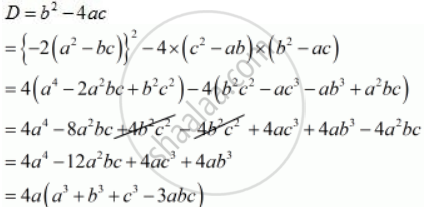Share

# If the Roots of the Equation (C2 – Ab) X2 – 2 (A2 – Bc) X + B2 – Ac = 0 in X Are Equal, Then Show that Either a = 0 Or A3 + B3 + C3 = 3abc - Mathematics

Course

#### Question

If the roots of the equation (c2 – ab) x2 – 2 (a2 – bc) x + b2 – ac = 0 in x are equal, then show that either a = 0 or a3 + b3 + c3 = 3abc

#### Solution

The given quadric equation is (c^2 - ab)x^2 - 2(a^2 - bc)x + (b^2 - ac) = 0

THen prove that either a = 0 or a^3 + b^3 + c^3 = 3abc

Here

a = (c^2 - ab), b = -2(a^2 - bc) " and " c = (b^2 - ac)

As we know that D = b^2 - 4ac

Putting the value of a = (c^2 - ab), b = -2(a^2 - bc) and c = (b^2 - ac)The given equation will have real roots, if D = 0

4a(a^3 + b^3 + c^3 - 3abc) = 0

a(a^3 +  b^3 + c^3 - 3abc) = 0

So , either

a = 0

or

(a^3 + b^3 + c^3 - 3abc) = 0

a^3 + b^3 + c^3 = 3abc

Hence, a = 0 or a^3 + b^3 + c^3 = 3abc

Is there an error in this question or solution?

#### APPEARS IN

RD Sharma Solution for Class 10 Maths (2018 (Latest))